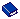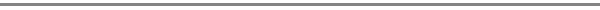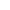OXI Tips and Tricks

Use this option to recalculate past daily statistics for a property. Select the calendar icon to the right of the From Date and the To Date fields and select the days to recalculate from the pop-up calendar. Select the OK button.

• Recalculate Summary Statistics - Use this utility to recalculate summary statistics.
• Recalculate Detailed Statistics - Use this utility to recalculate detailed statistics.
• Recalculate Revenue Bucket - Use this utility to recalculate revenue buckets  if you make changes to the association of transaction codes to revenue buckets.Also in This Section